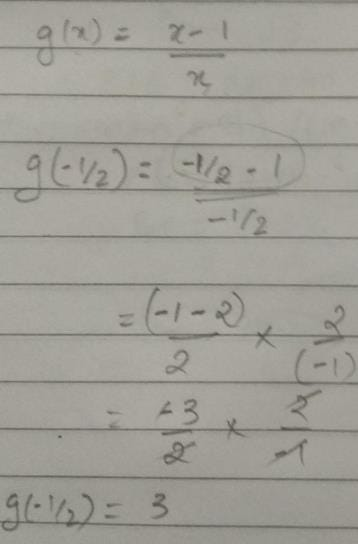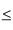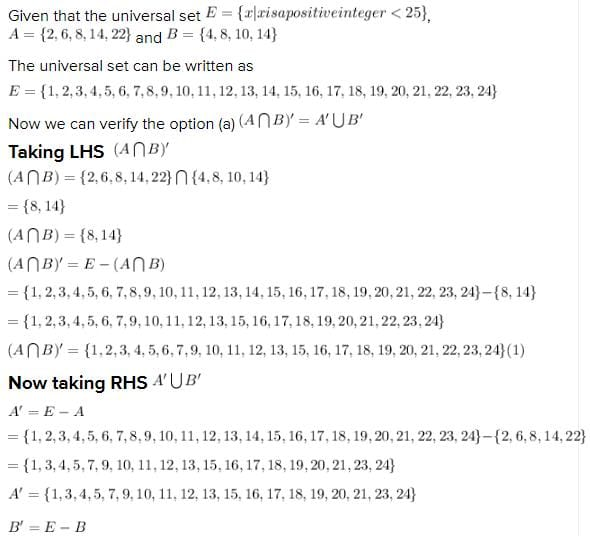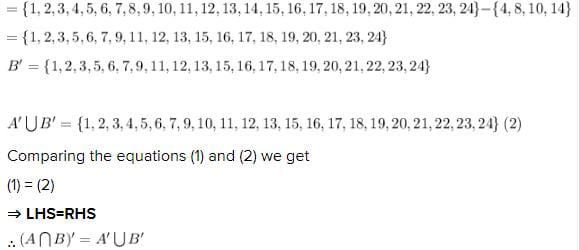Test: Sets- 2

# Test: Sets- 2

Test Description

## 40 Questions MCQ Test Quantitative Aptitude for CA CPT | Test: Sets- 2

Test: Sets- 2 for CA Foundation 2022 is part of Quantitative Aptitude for CA CPT preparation. The Test: Sets- 2 questions and answers have been prepared according to the CA Foundation exam syllabus.The Test: Sets- 2 MCQs are made for CA Foundation 2022 Exam. Find important definitions, questions, notes, meanings, examples, exercises, MCQs and online tests for Test: Sets- 2 below.
Solutions of Test: Sets- 2 questions in English are available as part of our Quantitative Aptitude for CA CPT for CA Foundation & Test: Sets- 2 solutions in Hindi for Quantitative Aptitude for CA CPT course. Download more important topics, notes, lectures and mock test series for CA Foundation Exam by signing up for free. Attempt Test: Sets- 2 | 40 questions in 40 minutes | Mock test for CA Foundation preparation | Free important questions MCQ to study Quantitative Aptitude for CA CPT for CA Foundation Exam | Download free PDF with solutions
 1 Crore+ students have signed up on EduRev. Have you?
Test: Sets- 2 - Question 1

### If f(x) = 1/1–x, f(–1) is

Test: Sets- 2 - Question 2

### If g(x) = (x–1)/x, g(–½) is

Detailed Solution for Test: Sets- 2 - Question 2Test: Sets- 2 - Question 3

### If f(x) = 1/1–x and g(x) = (x–1)/x, than fog(x) is

Test: Sets- 2 - Question 4

If f(x) = 1/1–x and g(x) = (x–1)/x, then g of(x) is

Test: Sets- 2 - Question 5

The function f(x) = 2x is

Test: Sets- 2 - Question 6

The range of the function f(x) = log10(1 + x) for the domain of real values of x when 0 ≤ x ≤9 is

Test: Sets- 2 - Question 7

The Inverse function f–1 of f(x) = 2x is

Test: Sets- 2 - Question 8

If f(x) = x+3, g(x) = x2, then fog(x) is

Test: Sets- 2 - Question 9

If f(x) = x+3, g(x) = x2 then f(x).g(x) is

Test: Sets- 2 - Question 10

The Inverse h–1 when h(x) = log10x is

Test: Sets- 2 - Question 11

For the function h(x) = 101+x the domain of real values of x where 0x9 , the range is

Test: Sets- 2 - Question 12

"Is smaller than" over the set of eggs in a box is

*Multiple options can be correct
Test: Sets- 2 - Question 13

"Is equal to" over the set of all rational numbers is

*Multiple options can be correct
Test: Sets- 2 - Question 14

"has the same father as" …… over the set of children

Test: Sets- 2 - Question 15

"is perpendicular to " over the set of straight lines in a given plane is

Test: Sets- 2 - Question 16

"is the reciprocal of" …….. over the set of non-zero real numbers is

*Multiple options can be correct
Test: Sets- 2 - Question 17

{(x, y)/x ∈ ×, y ∈ y, y = x} is

*Multiple options can be correct
Test: Sets- 2 - Question 18

{(x,y)/x + y = 2x where x and y are positive integers}, is

Test: Sets- 2 - Question 19

"Is the square of" over n set of real numbers is

Test: Sets- 2 - Question 20

If A has 32 elements, B has 42 elements and AUB has 62 elements, the number of elements in A ∩ B is

Test: Sets- 2 - Question 21

In a group of 20 children, 8 drink tea but not coffee and 13 like tea. The number of children drinking coffee but not tea is

Test: Sets- 2 - Question 22

The number of subsets of the sets {6, 8, 11} is

Test: Sets- 2 - Question 23

The sets V = {x / x+2=0}, R={x / x2+2x=0} and S = {x : x2+x–2=0} are equal to one another if x is equal to

Test: Sets- 2 - Question 24

If the universal set E = {x |x is a positive integer <25} , A = {2, 6, 8, 14, 22}, B = {4, 8, 10, 14} then

Detailed Solution for Test: Sets- 2 - Question 24Test: Sets- 2 - Question 25

If the set P has 3 elements, Q four and R two then the set P×Q×R contains

Test: Sets- 2 - Question 26

Given A = {2, 3}, B = {4, 5}, C = {5, 6} then A × (B ∩ C) is

Test: Sets- 2 - Question 27

A town has a total population of 50,000. Out of it 28,000 read the newspaper X and 23000 read Y while 4000 read both the papers. The number of persons not reading X and Y both is

Test: Sets- 2 - Question 28

If A = { 1, 2, 3, 5, 7} and B = {1, 3, 6, 10, 15}. Cardinal number of A~B is

Test: Sets- 2 - Question 29

Which of the diagram is graph of a function

Test: Sets- 2 - Question 30

At a certain conference of 100 people there are 29 Indian women and 23 Indian men. Out of these Indian people 4 are doctors and 24 are either men or doctors. There are no foreign doctors. The number of women doctors attending the conference is

Test: Sets- 2 - Question 31

Let A = {a, b}. Set of subsets of A is called power set of A denoted by P(A). Now n(P(A) is

Test: Sets- 2 - Question 32

Out of 2000 employees in an office 48% preferred Coffee (c), 54% liked (T), 64% used to smoke (S). Out of the total 28% used C and T, 32% used T and S and 30% preferred C and S, only 6% did none of these. The number having all the three is

Detailed Solution for Test: Sets- 2 - Question 32

Total = 2000  = 100 %

C = 48 %

T = 54 %

S = 64 %

C ∩ T = 28 %

T ∩ S = 32%

C ∩ S = 30 %

Having all three = C ∩ T ∩ S  = ?

None = 6 %

Total = C + T + S - C ∩ T  - T ∩ S - C ∩ S + C ∩ T ∩ S + None

=> 100 = 48 + 54 + 64 - 28 - 32 - 30 + C ∩ T ∩ S + 6

=> C ∩ T ∩ S = 18 %

18 % of 2000 = (18/100) * 2000 = 360

360 having all the three

Test: Sets- 2 - Question 33

Referred to the data of

Out of 2000 employees in an office 48% preferred Coffee (c), 54% liked (T), 64% used to smoke (S). Out of the total 28% used C and T, 32% used T and S and 30% preferred C and S, only 6% did none of these. The number having all the three is

Q. The number of employees having T and S but not C is

Test: Sets- 2 - Question 34

Referred to the data of

Out of 2000 employees in an office 48% preferred Coffee (c), 54% liked (T), 64% used to smoke (S). Out of the total 28% used C and T, 32% used T and S and 30% preferred C and S, only 6% did none of these. The number having all the three is

Q. The number of employees preferring only coffee is

Test: Sets- 2 - Question 35

If f(x) = x+3, g(x) = x2, then gof(x) is

Test: Sets- 2 - Question 36

If f(x) = 1/1–x, then f -1(x) is

Test: Sets- 2 - Question 37

Following set notations represent: – A ⊂ B; x∉ A; A ⊃B; {0}; A ⊄B

Test: Sets- 2 - Question 38

Represent the following sets in set notation: – Set of all alphabets in English language set of all odd integers less than 25 set of all odd integers set of positive integers x satisfying the equation x2 +5x+7=0 : -

Test: Sets- 2 - Question 39

Re-write the following sets in a set builder form: - A={a, e, i, o, u} B={1, 2, 3, 4 ….} C is a set of integers between –15 and 15.

Test: Sets- 2 - Question 40

If V={0, 1, 2, …9}, X={0, 2, 4, 6, 8}, Y={3, 5, 7} and Z={3 7} then Y ∪ Z, (V ∪ Y) ∩ X, (X ∪ Z) ∪ V  are respectively: –

## Quantitative Aptitude for CA CPT

67 docs|68 tests
 Use Code STAYHOME200 and get INR 200 additional OFF Use Coupon Code
Information about Test: Sets- 2 Page
In this test you can find the Exam questions for Test: Sets- 2 solved & explained in the simplest way possible. Besides giving Questions and answers for Test: Sets- 2, EduRev gives you an ample number of Online tests for practice

67 docs|68 tests